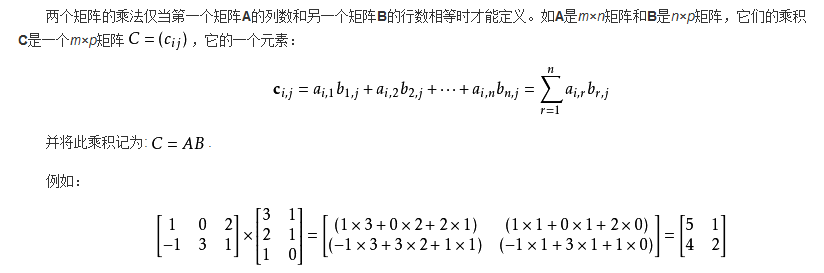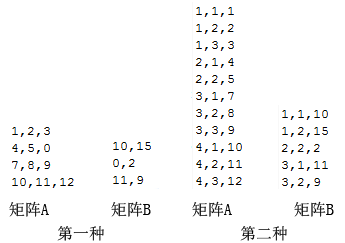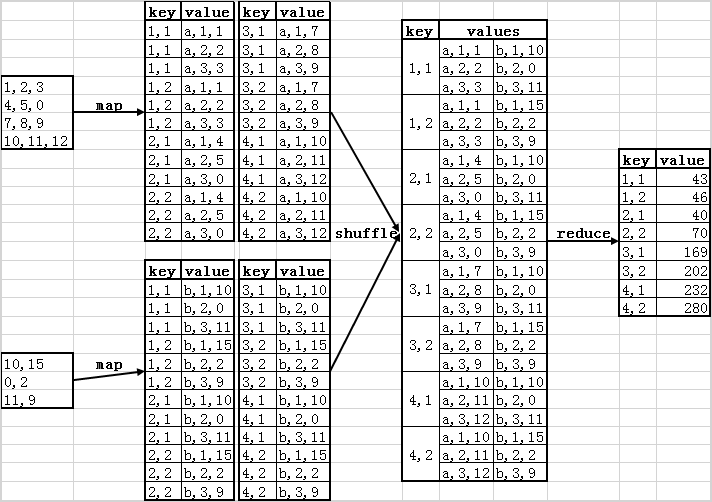• 对于一类特殊的二阶矩阵微分方程，给定特解的具体形式，利用向量比较方法解出了待定的系数矩阵，获得了一类矩阵微分方程的特解公式，推广了已有的结果。并用算例验证了结果的正确性。
• ## 二阶矩阵求导

千次阅读 2018-05-14 08:52:11
∵∇f(x)=2Ax+2b∵∇f(x)=2Ax+2b\because \nabla f(x)=2Ax+2b ∇f(x+αd)=2A(x+αd)+2b∇f(x+αd)=2A(x+αd)+2b\nabla f(x+\alpha d)=2A(x+\alpha d)+2b 又 ∵∂h(α)∂(α)=∇f(x+αd)∂(x+αd)∂α=∇f(x+αd)d=...


∵∇f(x)=2Ax+2b

∵

∇

f

(

x

)

=

2

A

x

+

2

b

$\because \nabla f(x)=2Ax+2b$

∇f(x+αd)=2A(x+αd)+2b

∇

f

(

x

+

α

d

)

=

2

A

(

x

+

α

d

)

+

2

b

$\nabla f(x+\alpha d)=2A(x+\alpha d)+2b$  又

∵∂h(α)∂(α)=∇f(x+αd)∂(x+αd)∂α=∇f(x+αd)d=0

∵

∂

h

(

α

)

∂

(

α

)

=

∇

f

(

x

+

α

d

)

∂

(

x

+

α

d

)

∂

α

=

∇

f

(

x

+

α

d

)

d

=

0

$\because \frac{ \partial h(\alpha)}{\partial (\alpha)} = \nabla f(x+\alpha d) \frac {\partial (x+\alpha d)}{\partial \alpha} =\nabla f(x+\alpha d)d=0$

∴

α

=

−

d

⊺

(

2

b

+

2

A

x

)

2

d

⊺

A

d

$\therefore \alpha=-\frac {d^\intercal (2b+2Ax)}{2d^\intercal Ad}$

=

−

d

⊺

∇

f

(

x

)

2

d

⊺

A

d

$=-\frac {d^\intercal \nabla f(x)}{2d^\intercal Ad}$
展开全文• 二阶矩阵相乘公式 上例中的C 11 =A 11 *B 11 +A 12 *B 21 +A 13 *B 31 =1*3+0*2+2*1=5、 C 12 =A 11 *B 12 +A 12 *B 22 +A 13 *B 32 =1*1+0*1+2*0=1 分析  因为分布式计算的特点，需要找到...
二阶矩阵相乘公式上例中的C11=A11*B11+A12*B21+A13*B31=1*3+0*2+2*1=5、C12=A11*B12+A12*B22+A13*B32=1*1+0*1+2*0=1

分析
因为分布式计算的特点，需要找到相互独立的计算过程，以便能够在不同的节点上进行计算而不会彼此影响。根据矩
阵乘法的公式，C中各个元素的计算都是相互独立的，即各个cij在计算过程中彼此不影响。这样的话，在Map阶段可
以把计算所需要的元素都集中到同一个key中，然后，在Reduce阶段就可以从中解析出各个元素来计算cij。  另外，
以a11为例，它将会在c11、c12...c1p的计算中使用，以b11为例，它将会在c11、c21...cm1的计算中使用，也就是说，在Map阶段，当我们从HDFS取出一行记录时，如
果该记录是A的元素，则需要存储成p个<key, value>对，并且这p个key互不相同；如果该记录是B的元素，则需要存
储成m个<key, value>对，同样的，m个key也应互不相同；但同时，用于存放计算cij的ai1、ai2……ain和b1j、
b2j……bnj的<key, value>对的key应该都是相同的，这样才能被传递到同一个Reduce中。

设计
普遍有一个共识是：数据结构+算法=程序，所以在编写代码之前需要先理清数据存储结构和处理数据的算法。
Map阶段
在Map阶段，需要做的是进行数据准备。把来自矩阵A的元素aij，标识成p条<key, value>的形式，key="i,k",（其中
k=1,2,...,p），value="A,j,Aij"；把来自矩阵B的元素bij，标识成m条<key, value>形式，key="k,j"（其中
k=1,2,...,m），value="B,i,Bij"。  经过处理，用于计算cij需要的a、b就转变为有相同key（"i,j"）的数据对，通过value
中"A"、"B"能区分元素是来自矩阵A还是矩阵B，以及具体的位置（在矩阵A的第几列，在矩阵B的第几行）。
Shuffle阶段
Reduce阶段
在Reduce阶段，有两个问题需要解决：
a. 当前的<key, list(value)>对是为了计算矩阵C的哪个元素？因为map阶段对数据的处理，key（i,j）中的数据对，就
是其在矩阵C中的位置，第i行j列。
b. list中的每个value是来自矩阵A或矩阵B的哪个位置？这个也在map阶段进行了标记，对于value（x,y,z），只需要找
到y相同的来自不同矩阵（即x分别为A和B）的两个元素，取z相乘，然后加和即可。
矩阵的两种表示方式
矩阵常用的两种表示方式，第一种是原始的表示方式，第二种是稀疏矩阵（只存储非0的元素）的表示方式。
第一种：使用最原始的表示方式，相同行内不同列数据通过","分割，不同行通过换行分割；
第二种：通过行列表示法，即文件中的每行数据有三个元素通过分隔符分割，第一个元素表示行，第二个元素表示
列，第三个元素表示数据。这种方式对于可以不列出为0的元素，即可以减少稀疏矩阵的数据量。编写代码：
第一种数据结构
查看源数据：

[hadoop@master ~]$hadoop fs -cat /user/hdfs/matrix/B 10,15 0,2 11,9 [hadoop@master ~]$ hadoop fs -cat /user/hdfs/matrix/A
1,2,3
4,5,0
7,8,9
10,11,12


MartrixMultiply：

package com.oner.mr.matrix;

import java.io.IOException;
import java.util.HashMap;
import java.util.Iterator;
import java.util.Map;

import com.oner.mr.util.HdfsDAO;

public class MartrixMultiply {

public static class MatrixMapper extends
Mapper<LongWritable, Text, Text, Text> {

private String flag;// A or B;
private int m = 4;// 矩阵A的行数
private int p = 2;// 矩阵B的列数
private int rowIndexA = 1; // 矩阵A，当前在第几行
private int rowIndexB = 1; // 矩阵B，当前在第几行

@Override
protected void setup(Context context) throws IOException,
InterruptedException {
FileSplit split = (FileSplit) context.getInputSplit();
flag = split.getPath().getName();// // 得到读取的矩阵名称
}

@Override
protected void map(LongWritable k1, Text v1, Context context)
throws IOException, InterruptedException {
// 切分每行数据
String[] fields = MainRun.DELIMITER.split(v1.toString());

if (flag.equals("A")) {// 如果读的是矩阵A，则fields格式为{1,2,3},数组长度为3
for (int k = 1; k <= p; k++) {// p表示矩阵B的列数
Text key = new Text(rowIndexA + "," + k);//
for (int j = 0; j < fields.length; j++) {// j代表矩阵A的当前列,fields.length表示矩阵A的列数，等于矩阵B的行数
Text value = new Text("A," + (j + 1) + "," + fields[j]);// v的值为
context.write(key, value);// 输出的数据格式key为(i,k),value为(A,j,Aij)。
System.out.println(key.toString() + "  "
+ value.toString());
}

}
rowIndexA++; // 每执行一次map方法，矩阵向下移动一行

} else if (flag.equals("B")) {// 如果读的是B,fields的格式为{10,15},数组长度为2
for (int k = 1; k <= m; k++) {// m表示矩阵A的行数
for (int j = 0; j < fields.length; j++) {// fields.length表示矩阵B的列数
Text key = new Text(k + "," + (j + 1));
Text value = new Text("B:" + rowIndexB + ","
+ fields[j]);
context.write(key, value);// 输出的数据格式key为(k,j),value为(B,i,Bij)。
System.out.println(key.toString() + "  "
+ value.toString());
}
}
rowIndexB++;// 每执行一次map方法，矩阵向下移动一行
}
}

}

public static class MatrixReducer extends
Reducer<Text, Text, Text, LongWritable> {
@Override
protected void reduce(Text key, Iterable<Text> values, Context context)
throws IOException, InterruptedException {
Map<String, String> mapA = new HashMap<String, String>();
Map<String, String> mapB = new HashMap<String, String>();

System.out.print(key.toString() + ":");

for (Text value : values) {
String val = value.toString();
System.out.print("(" + val + ")");

if (val.startsWith("A")) {
String[] kv = MainRun.DELIMITER.split(val.substring(2));// 得到A,j,Aij中的j,Aij
mapA.put(kv, kv);// 将j作为key,Aij作为value存入mapA

// System.out.println("A:" + kv + "," + kv);

} else if (val.startsWith("B")) {
String[] kv = MainRun.DELIMITER.split(val.substring(2));// 得到B,j,Bij中的i,Bij
mapB.put(kv, kv);// 将i作为key,Bij作为value存入mapB

// System.out.println("B:" + kv + "," + kv);
}
}

long result = 0;
Iterator<String> mkeys = mapA.keySet().iterator();// 得到mapA所有的键集合
while (mkeys.hasNext()) {
String mkey = mkeys.next();
if (mapB.get(mkey) == null) {// 因为mkey取的是mapA的key集合，所以只需要判断mapB是否存在即可。
continue;
}
result += Long.parseLong(mapA.get(mkey))
* Long.parseLong(mapB.get(mkey));
}
context.write(key, new LongWritable(result));
System.out.println();

// System.out.println("C:" + key.toString() + "," + result);

}
}

public static void run(Map<String, String> path) throws IOException,
ClassNotFoundException, InterruptedException {

Configuration conf = new Configuration();

String input = path.get("input");
String input1 = path.get("input1");
String input2 = path.get("input2");
String output = path.get("output");

HdfsDAO hdfs = new HdfsDAO(MainRun.HDFS, conf);
hdfs.rmr(input);
hdfs.mkdirs(input);
hdfs.copyFile(path.get("A"), input1);
hdfs.copyFile(path.get("B"), input2);

Job job = Job.getInstance(conf);
job.setJarByClass(MainRun.class);

job.setOutputKeyClass(Text.class);
job.setOutputValueClass(Text.class);

job.setMapperClass(MatrixMapper.class);
job.setReducerClass(MatrixReducer.class);

job.setInputFormatClass(TextInputFormat.class);
job.setOutputFormatClass(TextOutputFormat.class);

FileInputFormat.setInputPaths(job, new Path(input1), new Path(input2));// 加载2个输入数据集
FileOutputFormat.setOutputPath(job, new Path(output));

job.waitForCompletion(true);
}
}


MainRun：

package com.oner.mr.matrix;

import java.io.IOException;
import java.util.HashMap;
import java.util.Map;
import java.util.regex.Pattern;

/*
* 驱动程序
*/
public class MainRun {

public static final String HDFS = "hdfs://master:9000";
public static final Pattern DELIMITER = Pattern.compile("[\t,]");

public static void main(String[] args) throws ClassNotFoundException,
IOException, InterruptedException {
martrixMultiply();
}

private static void martrixMultiply() throws ClassNotFoundException,
IOException, InterruptedException {
Map<String, String> path = new HashMap<String, String>();
path.put("input", HDFS + "/user/hdfs/matrix");// HDFS的目录
path.put("input1", HDFS + "/user/hdfs/matrix/A");
path.put("input2", HDFS + "/user/hdfs/matrix/B");
path.put("output", HDFS + "/user/hdfs/matrix/output");

MartrixMultiply.run(path);
}

}


查看结果：

[hadoop@master ~]$hadoop fs -cat /user/hdfs/matrix/output/part-r-00000 1,1 43 1,2 46 2,1 40 2,2 70 3,1 169 3,2 202 4,1 232 4,2 280 绘图演示结果：第二种数据结构 MainRun： package com.oner.mr.sparsematrix; import java.io.IOException; import java.util.HashMap; import java.util.Map; import java.util.regex.Pattern; /* * 驱动程序 */ public class MainRun { public static final String HDFS = "hdfs://master:9000"; public static final Pattern DELIMITER = Pattern.compile("[\t,]"); public static void main(String[] args) throws ClassNotFoundException, IOException, InterruptedException { sparseMartrixMultiply(); } private static void sparseMartrixMultiply() throws ClassNotFoundException, IOException, InterruptedException { Map<String, String> path = new HashMap<String, String>(); path.put("A", "/home/hadoop/logfile/matrix2/A.csv");// 本地的数据文件 path.put("B", "/home/hadoop/logfile/matrix2/B.csv"); path.put("input", HDFS + "/user/hdfs/matrix2");// HDFS的目录 path.put("input1", HDFS + "/user/hdfs/matrix2/A"); path.put("input2", HDFS + "/user/hdfs/matrix2/B"); path.put("output", HDFS + "/user/hdfs/matrix2/output"); MartrixMultiply.run(path); } }  MartrixMultiply： package com.oner.mr.sparsematrix; import java.io.IOException; import java.util.HashMap; import java.util.Iterator; import java.util.Map; import org.apache.hadoop.conf.Configuration; import org.apache.hadoop.fs.Path; import org.apache.hadoop.io.LongWritable; import org.apache.hadoop.io.Text; import org.apache.hadoop.mapreduce.Job; import org.apache.hadoop.mapreduce.Mapper; import org.apache.hadoop.mapreduce.Reducer; import org.apache.hadoop.mapreduce.lib.input.FileInputFormat; import org.apache.hadoop.mapreduce.lib.input.FileSplit; import org.apache.hadoop.mapreduce.lib.input.TextInputFormat; import org.apache.hadoop.mapreduce.lib.output.FileOutputFormat; import org.apache.hadoop.mapreduce.lib.output.TextOutputFormat; import com.oner.mr.util.HdfsDAO; public class MartrixMultiply { public static class SparseMatrixMapper extends Mapper<LongWritable, Text, Text, Text> { private String flag;// A or B; private int m = 4;// 矩阵A的行数 private int p = 2;// 矩阵B的列数 @Override protected void setup(Context context) throws IOException, InterruptedException { FileSplit split = (FileSplit) context.getInputSplit(); flag = split.getPath().getName();// // 得到读取的矩阵名称 } @Override protected void map(LongWritable k1, Text v1, Context context) throws IOException, InterruptedException { // 切分每行数据 String[] fields = MainRun.DELIMITER.split(v1.toString()); if ("A".equals(flag)) { for (int i = 1; i <= p; i++) { context.write(new Text(fields + "," + i), new Text("A," + fields + "," + fields)); } } else if ("B".equals(flag)) { for (int i = 1; i <= m; i++) { context.write(new Text(i + "," + fields), new Text("B," + fields + "," + fields)); } } } } public static class SparseMatrixReducer extends Reducer<Text, Text, Text, LongWritable> { @Override protected void reduce(Text key, Iterable<Text> values, Context context) throws IOException, InterruptedException { Map<String, String> mapA = new HashMap<String, String>(); Map<String, String> mapB = new HashMap<String, String>(); for (Text value : values) { String val = value.toString(); if (val.startsWith("A")) { String[] kv = MainRun.DELIMITER.split(val.substring(2));// 得到A,j,Aij中的j,Aij mapA.put(kv, kv);// 将j作为key,Aij作为value存入mapA } else if (val.startsWith("B")) { String[] kv = MainRun.DELIMITER.split(val.substring(2));// 得到B,j,Bij中的i,Bij mapB.put(kv, kv);// 将i作为key,Bij作为value存入mapB } } long result = 0; // 可能在mapA中存在在mapB中不存在的key，或相反情况 // 因为，数据定义的时候使用的是稀疏矩阵的定义 // 所以，这种只存在于一个map中的key，说明其对应元素为0，不影响结果 Iterator<String> mkeys = mapA.keySet().iterator();// 得到mapA所有的键集合 while (mkeys.hasNext()) { String mkey = mkeys.next(); if (mapB.get(mkey) == null) {// 因为mkey取的是mapA的key集合，所以只需要判断mapB是否存在即可。 continue; } result += Long.parseLong(mapA.get(mkey)) * Long.parseLong(mapB.get(mkey)); } context.write(key, new LongWritable(result)); } } public static void run(Map<String, String> path) throws IOException, ClassNotFoundException, InterruptedException { Configuration conf = new Configuration(); String input = path.get("input"); String input1 = path.get("input1"); String input2 = path.get("input2"); String output = path.get("output"); HdfsDAO hdfs = new HdfsDAO(MainRun.HDFS, conf); hdfs.rmr(input); hdfs.mkdirs(input); hdfs.copyFile(path.get("A"), input1); hdfs.copyFile(path.get("B"), input2); Job job = Job.getInstance(conf); job.setJarByClass(MainRun.class); job.setOutputKeyClass(Text.class); job.setOutputValueClass(Text.class); job.setMapperClass(SparseMatrixMapper.class); job.setReducerClass(SparseMatrixReducer.class); job.setInputFormatClass(TextInputFormat.class); job.setOutputFormatClass(TextOutputFormat.class); FileInputFormat.setInputPaths(job, new Path(input1), new Path(input2));// 加载2个输入数据集 FileOutputFormat.setOutputPath(job, new Path(output)); job.waitForCompletion(true); } }  打成jar包运行：hadoop jar matrix 查看结果： [hadoop@master matrix2]$ hadoop fs -cat /user/hdfs/matrix2/output/part-r-00000
1,1	43
1,2	46
2,1	40
2,2	70
3,1	169
3,2	202
4,1	232
4,2	280


展开全文Hadoop
• 优化算法的问题在于如何从XkX_kXk​更新到Xk+1X_{k+1}Xk+1​、确定步长公式（详见 基于二阶矩阵的优化问题（二））和判定迭代终止的条件（详见 于二阶矩阵的优化问题（三））是我们需要在不精确搜索中解决的问题。...


基于二阶矩阵的优化问题（一）线搜索策略
非精确线搜索更新

X

k

+

1

X_{k+1}

线搜索策略最速下降+牛顿法启发
修正牛顿法特征值修正（eigenvalue modification）谱分解
单位增量法
拟牛顿法

非精确线搜索更新

X

k

+

1

X_{k+1}

优化算法的问题在于如何从

X

k

X_k

更新到

X

k

+

1

X_{k+1}

、确定步长公式详见 基于二阶矩阵的优化问题（二）和判定迭代终止的条件（详见 于二阶矩阵的优化问题（三））是我们需要在不精确搜索中解决的问题。 我们有两种方法来解决这个问题： 1.线搜索策略 2.信任域策略
线搜索策略
我们选择一个下降方向来使得lost function慢慢迭代至最小值。

X

k

+

1

=

X

k

+

α

k

p

k

X_{k+1}=X_k+α_kp_k

这是

X

k

+

1

X_{k+1}

的更新公式，可以看到，我们需要知道其

α

k

α_k

和

p

k

p_k

的值才能进行迭代（

α

k

α_k

代表了步长、

p

k

p_k

代表下降方向），第一种方法就是

p

k

p_k

走一个最速下降方向（负梯度），而

α

k

α_k

走一个极其猥琐的距离，但这种方法在函数比较诡异时效果较差（如rosenbrock函数），但在某些复杂问题时，我们还是使用最速下降来解决问题，详见 大规模的优化问题（一）。
最速下降+牛顿法
让我们来列一下牛顿方向的式子：

f

(

x

k

+

p

)

≈

f

k

+

p

T

∇

f

k

+

1

2

p

T

∇

2

f

k

p

f(x_k+p)\approx f_k+p^T\nabla f_k+\frac{1}{2}p^T\nabla^2f_kp

在这里，我们要假设这里的二次型（

∇

2

f

k

\nabla^2 f_k

）是正定的，这是函数的局部二次型构型就是碗状的，我们可以求出这里的pk（下降方向）：

p

K

N

=

−

(

∇

2

f

k

)

−

1

∇

f

k

p^N_K=-(\nabla^2f_k)^{-1}\nabla f_k

;

这边的

p

K

N

p^N_K

就是这里的局部二次型的顶点，在牛顿法中，我们可以一步就走到这个点。 ok，这个问题的理论情况是这样的，但在实际问题中，我们不可能去求一个hessian矩阵的逆矩阵，所以，我们把

1

/

(

∇

2

f

k

)

1/(\nabla^2f_k)

移到等式的左边，变成：

p

K

N

∗

(

∇

2

f

k

)

=

−

∇

f

k

p^N_K*(\nabla^2f_k)=-\nabla f_k

，

这个等式实际上就等于Ax=b的形式，这边我们只需要求解一个线性方程组即可。 以上为牛顿法的基础理论，下面看一下牛顿法的实现步骤：

Created with Raphaël 2.2.0

开始

最速下降

▽fk<阈值

转到Newton

yes

no

可以看到，牛顿法的意义在于足够靠近真解时的快速收敛，我们先走几部最速下降法，使得足够靠近阈值，后再启动牛顿法，会有较好的效果,即保证了算法的效率，有保证了算法的鲁棒性。
下面是最速下降法+牛顿法matlab实现的代码：
function [x1] = min_hybrid(func, dfunc, d2func ,x, MAX_IT ,eps, threshold)
err = 10.0;
steps = 0;
alpha = 1.0;
//最速下降法
while (err > threshold)//此处阈值需要调参
f = func(x);
g = dfunc(x);
p = -g;
step=step+1;
alpha = backtracking(@func, x, f, g, p, 1e-4, 0.8, alpha * 2.0);
x = x + alpha * p;
if (steps > MAX_IT)
break;
end
error = norm(g);
end
//牛顿法
while (error > eps)
f = func(x);
g = dfunc(x);
G = d2func(x);
p = -G\g;
x = x + p;
step=step+1;
if (steps > MAX_IT)
break;
end
error = norm(g);
end
x1=x;
end

function alpha = backtracking(func, x, f, df, p, c, rho, alpha0)
alpha = alpha0;
while (func(x(1) + alpha * p(1), x(2) + alpha * p(2)) > f + c * alpha * df' * p)
alpha = alpha * rho;
end
end

其中func、gfunc和hess需要自行解析计算，func的接口只有x，gfunc和hess没有输入参数。（这边要注意，使用wolfe条件时，alpha可以恒取1）
启发
牛顿法实际上是在改进真解，用少量次数的迭代即可实现一阶方法上千次的迭代效果，首先系数矩阵必须要正定，否则牛顿法不能保证收敛。
修正牛顿法
牛顿法最大的问题在于，系数矩阵必须要正定，那么如果系数矩阵不正定，则将

B

k

B_k

改成正定（modification），下面是修改系数矩阵的算法框架：

初值

x

0

x_0

for k=0,1,2,…         令

B

k

=

∇

2

f

k

+

E

k

B_k=\nabla^2 f_k+E_k

,其中

E

k

E_k

为修正项，使得

B

k

B_k

充分正定。         求解

B

k

p

k

=

−

∇

f

k

B_kp_k=-\nabla f_k

x

k

+

1

=

x

k

+

α

k

p

k

x_{k+1}=x_k+α_kp_k

（其中

α

k

α_k

在wolfe条件时可以取1） end

好了，现在我们需要解决四件事，就可以实现修正牛顿法的运用： 1.判定

∇

2

f

k

\nabla^2 f_k

是否正定； 2.解方程组

B

k

p

k

=

−

∇

f

k

B_kp_k=-\nabla f_k

； 3.如何构建

E

k

E_k

； 4.如何使得

∣

∣

E

k

∣

∣

\vert\vert E_k\vert\vert

最小；
特征值修正（eigenvalue modification）
谱分解
首先我们先对对称矩阵

B

k

B_k

做正交分解（householder等），得到正交阵Q，和对角阵V，然后就可以得到

B

k

B_k

的所有特征值，我们知道，正定矩阵所有的特征值都是充分大的正数，即

λ

m

i

n

>

δ

>

>

e

p

s

>

0

λ_{min}>δ>>eps>0

，当出现负的特征值时，我们添加一个修正项

E

k

E_k

使得特征值大于等于δ。此时的

∣

∣

E

k

∣

∣

\vert\vert E_k\vert\vert

的Frobenius范数最小。 即用Matlab中的eig()函数求出特征值后，直接对特征值修改即可。
单位增量法
谱分解的问题在于，其真正改变的是特征值，所以对

B

k

B_k

的改动是比较大的，当我们不希望

B

k

B_k

发生很大的变动时，我们就使用单位增量法。单位增量法直接对

B

k

B_k

添加一个修正项

E

k

I

E_kI

，使得其正定。 首先，我们对

B

k

B_k

进行cholesky分解：

B

k

=

L

D

L

T

B_k=LDL^T

L是对角线均为1的下三角阵，D为对角阵，若

B

k

B_k

不定，D的对角元

d

i

i

d_{ii}

会过大。 下面是单位增量法的Matlab代码：
function[L,D]=modification(A,delta,beta)
if(norm(A-A')>eps
return;
end
n=size(A,1);
d=zero(n,1);
L=zero(n);
C=zero(n);
theta=zeros(n,1);
for j = 1:n
C(j,j) = A(j,j)-sum(d(1:j-1)'.*L(j,1:j-1).^2);
for i = j+1:n
C(i,j) = A(i,j)-sum(d(1:j-1)'.*L(i,1:j-1).*L(j,1:j-1));
absjj=abs(C(i,j));
if theta < abs(C(i,j))
theta = abs(C(i,j));
end
end
d(j) = max([abs(C(j,j)), (theta/beta)^2, \delta]);
for i = j+1:n
L(i,j) = C(i,j)/d(j);
end
L(j,j) = 1.0;
end
D=diag(d);
end

单位增量法得到的

B

k

B_k

和

B

k

+

E

k

B_k+E_k

在形式上很接近，但其特征值完全不同，所以两种方法各有优势，可根据情况自行选择。
拟牛顿法
如果你的数据维数过大或者无法承受计算二阶矩阵的消耗，这边也可以使用拟牛顿法来计算下降方向，当然拟牛顿法也有其缺陷，下面我们来分析一下。 首先我们介绍一下割线法，用弦的斜率近似代替目标函数的切线斜率，下面给出割线法的公式

x

k

+

1

=

x

k

−

f

(

x

k

)

∗

x

k

−

1

−

x

k

f

(

x

k

−

1

)

−

f

(

x

k

)

x_{k+1}=x_k-f(x_k)*\frac{x_{k-1}-x_k}{f(x_{k-1})-f(x_k)}

这在本质上是计算函数的数值微分，但这种方法在接近收敛时会出现数值不稳定的情况，当接近收敛时，舍入误差占比变大，数值会发生很大的起伏变化。 由此我们可以推导出拟牛顿法的公式

x

k

+

1

=

x

k

−

∇

f

(

x

k

)

∗

x

k

−

1

−

x

k

∇

f

(

x

k

−

1

)

−

∇

f

(

x

k

)

x_{k+1}=x_k-\nabla f(x_k)*\frac{x_{k-1}-x_k}{\nabla f(x_{k-1})-\nabla f(x_k)}

这里不在需要计算其hessian矩阵，由于hessian矩阵是

n

2

n^2

级别的，拟牛顿法对于大规模问题来说是非常节约资源的方法。
于是我们知道了

∇

2

f

(

x

k

)

∗

(

x

k

+

1

−

x

k

)

≈

∇

f

x

+

1

−

∇

f

k

\nabla ^2f(x_k)*(x_{k+1}-x_k)\approx\nabla f_{x+1}-\nabla f_k

现在的情况与牛顿发不同，

∇

f

(

x

k

)

\nabla f(x_k)

并不知道，这是一个不定方程组:

B

k

+

1

∗

s

k

=

y

k

B_{k+1}*s_k=y_k

这里的

B

k

+

1

B_{k+1}

是

n

2

n^2

阶的矩阵，而我们只有n个方程，下面有SR1、BFGS等方法来求解

B

k

+

1

B_{k+1}

。 详见 拟牛顿法的下降方向计算（一） 此时，局部二次型的

p

K

N

p_K^N

方程变为

p

K

N

=

−

(

B

k

)

−

1

∇

f

k

p^N_K=-(B_k)^{-1}\nabla f_k

;

这里还有一条路，就是去构建

(

B

k

)

−

1

(B_k)^{-1}

,这时我们不需要再去求解线性方程组（这里设

H

k

=

(

B

k

)

−

1

H_k=(B_k)^{-1}

），这里

B

k

B_k

=I时，就是最速下降。

p

k

=

−

H

k

∗

∇

f

k

p_k=-H_k*\nabla f_k

这里有拟BFGS等方法来求解

H

k

H_k

,详见 拟牛顿法的下降方向计算（二）
github代码地址
展开全文算法 matlab 深度学习 人工智能
• 基于微分方程组理论和矩阵理论,采用按列比较方法和待定矩阵方法,给出了非齐次项为二次多项式与指数函数乘积的一类三维二阶常系数线性微分方程组的特解公式。对特殊情况进行了讨论,并通过算例验证了微分方程组特解...
• 基于微分方程组理论和矩阵理论，采用待定矩阵方法和按列比较方法，给出了非齐次项为三角函数与指数函数乘积的一类三维二阶常系数线性微分方程组的特解公式，对3种特殊情况进行了讨论，并通过算例验证了微分方程组特...
• 基于二阶矩阵的优化问题（二）非精确搜索确定步长沃尔夫（wolfe）条件Armijo条件曲率下降条件强沃尔夫（wolfe）条件Goldstein条件回溯法（backtracking） 非精确搜索确定步长 在之前的文章中，我们已经讨论了如何从...


基于二阶矩阵的最优化问题（二）
非精确搜索确定步长沃尔夫（wolfe）条件Armijo条件曲率下降条件
强沃尔夫（wolfe）条件Goldstein条件回溯法（backtracking）

非精确搜索确定步长
在之前的文章中，我们已经讨论了如何从

X

k

X_k

更新到

X

k

+

1

X_{k+1}

，这篇文章我们主要不精确搜索中确定步长的方法
沃尔夫（wolfe）条件
wolfe条件分为Armijo条件和曲率下降条件，只要函数f有下界，所求的α就满足wolfe和强wolfe条件。
Armijo条件
Armijo条件的优点在于其计算的代价很小，只需要常数阶的计算就可以得到有效的α，下面是Armijo条件的公式：

f

(

x

k

+

α

p

k

)

≤

f

(

x

k

)

+

c

1

α

∇

f

k

T

p

k

,

c

1

∈

(

0

,

1

)

f(x_k+αp_k)\le f(x_k)+c_1α\nabla f_k^Tp_k,c_1\in (0,1)

我们用一张图来直观解释这个条件：这里的红线表示α可以取到的区间，我们发现，Armijo条件的α会取到充分小的值，这对于迭代来说是非常不利的，Armijo条件无法保证充分下降，这会导致函数无法收敛。这里的

c

1

c_1

（学习率）一般取

1

0

−

4

10^{-4}

。
曲率下降条件
曲率下降条件需要计算一个梯度，这里给出其公式：

∇

f

(

x

k

+

α

p

k

)

T

p

k

≥

c

2

∇

f

k

T

p

k

,

c

2

∈

(

c

1

,

1

)

\nabla f(x_k+αp_k)^Tp_k\geq c_2\nabla {f_k}^Tp_k,c_2\in (c_1,1)

在不精确计算中，这个计算的代价是很大的，但曲率下降条件为α提供了一个范围，其保证了α不会取到充分小的值，保证了充分下降，这里我们也用一张图来解释曲率下降条件：这边的红线表示α取不到的区间，在开始时

∇

f

k

T

p

k

\nabla {f_k}^Tp_k

是小于0的，移动到中间点后，其梯度会比出发时大一点,我们可以看到，

c

2

c_2

这个参数约束了α取到充分小的值，至少在每一步都会有一个微小的下降，我们联立Armijo条件和曲率下降条件就可以得到充分下降的α，在牛顿法中,一般

c

2

≈

1

c_2\approx1

;在CG法中

c

2

≈

0.1

c_2\approx0.1

。
强沃尔夫（wolfe）条件
强wolfe条件相对于wolfe条件来说就是做了一个左右对称，即：

∣

∇

f

(

x

k

+

α

p

k

)

T

p

k

∣

≥

c

2

∣

∇

f

k

T

p

k

∣

,

c

2

∈

(

c

1

,

1

)

\vert\nabla f(x_k+αp_k)^Tp_k\vert\geq c_2\vert\nabla {f_k}^Tp_k\vert,c_2\in (c_1,1)

强wolfe条件中参数的选取和wolfe公式中相同，我们取

c

1

≈

1

0

−

4

c_1\approx10^{-4}

,在牛顿法中,一般

c

2

≈

1

c_2\approx1

;在CG法中

c

2

≈

0.1

c_2\approx0.1

。
Goldstein条件
之前的wolfe条件（尤其是强wolfe条件）需要计算梯度，这个代价非常的大，但不计算梯度有可能导致算法不收敛，那么Goldstein条件可以解决这个问题，下面给出Goldstein条件的公式：

f

k

+

(

1

−

c

)

α

k

∇

f

k

T

p

k

≤

f

(

x

k

+

α

k

p

k

)

≤

f

k

+

c

α

k

∇

f

k

T

p

k

f_k+(1-c)α_k\nabla f_k^Tp_k\le f(x_k+α_kp_k)\le f_k+cα_k\nabla f_k^Tp_k

(

0

<

c

<

0.5

)

(0\lt c\lt0.5)下面有一张图来直观解释Goldstein条件：
这里的红线表示α可以取到的区间，我们发现，Goldstein条件抛弃了局部极值点，但是其计算方便，只需要常数阶计算就可以计算出α（和Armijo条件相似），且Goldstein条件可以保证充分下降。
回溯法（backtracking）
之前我们提到，Armijo条件的缺点在于α的值会取到充分小，那么先将α取较大的值，然后乘以一个系数p（小于1），使其慢慢下降就可以避免α的值取到充分小，在实际应用中基本没有问题。 回溯法就是基于这个思想创造的：

Created with Raphaël 2.2.0

取一个较大的α值

是否满足Armijo条件

αk=α

α=p*α

yes

no

这里的

α

ˉ

>

0

,

p

∈

(

0

,

1

)

,

c

∈

(

0

,

1

)

\bar α>0,p\in(0,1),c\in(0,1)

，除非有非常强烈的条件提示我们需要用wolfe条件（如多尺度问题），我们一般使用回溯法来编写程序。 在p->1时，找到的α比较长，但找的比较慢；在p->0时，找到的α比较短，但找的比较快，所以需要结合不同实际问题进行调参。
下面是我在github的wolfe和回溯法的MATLAB实现代码：代码点击此处
 while(num_iter)// wolfe方向（Matlab）
if~(feval(func,x0+alpha*dk)<=func(x0)+rho*alpha*feval(gfunc,x0)'*dk)
b=alpha;
alpha=(alpha+a)/2;
continue;
end
if~(feval(gfunc,x0+alpha*dk)'*dk>=sigma*feval(gfunc,x0)'*dk)
a=alpha;
alpha=min([2*alpha,(b+alpha)/2]);
continue;
end
break;
end

//回溯法(Matlab)
alpha_k=10;//该参数可调（初始alpha_k应该较大）
p=0.5;
epa=10-6;
while(alpha>epa)
if~(feval(func,x0+alpha*dk)>func(x0)+rho*alpha*feval(gfunc,x0)'*dk)
alpha=alpha*p;
end
end


展开全文机器学习 算法 深度学习 人工智能
• 二阶矩阵 三阶矩阵 矩阵 对于考研以及学习矩阵论具有重要意义
• 采用复高斯展开法和维格纳分布函数(WDF)，推导出了截断光束的二阶矩阵通过大气湍流的传输公式。研究表明，将硬边光阑的复高斯展开函数引入z=0平面处的WDF中，能够避免截断光束二阶矩的积分发散问题，得到z=0平面处...
• 矩阵A=(a11a12a13a21a22a23a31a32a33)A = \begin{pmatrix} a_{11} &amp;amp; a_{12} &amp;amp; a_{13}\\ a_{21}&amp;amp; a_{22} &amp;amp; a_{23}\\ a_{31}&amp;amp; a_{32} &amp;amp;...行列式
• 详细介绍了雅可比矩阵逆解的思路与方法，英文版，值得研究
•技术
• 首先我们来认识一下主要的分块矩阵的矩阵的类型有以下几种： ...我们先来看主对角线分块矩阵的逆矩阵公式规律： 主对角线分块矩阵的分块矩阵位置不变，主对角线上的分块矩阵分别添上（-1）求各自的矩阵，
• 问题及代码： /* ...*All rights reserved....*文件名称：matrix.cpp *作 者：单昕昕 *完成日期：2014年12月4日 *版 本 号：v1.0 ...*程序输出：输出这两个二阶矩阵相乘的结果。 */ #include using namespc++ 矩阵
• 转置矩阵： 将矩阵的行列互换得到的新矩阵称为转置矩阵，转置矩阵的行列式不变。 例如， ， 。 如果阶方阵和它的转置相等 ，即，则称矩阵为对称矩阵。 如果，则称矩阵为反对称矩阵。 正交矩阵： 如果：AAT=E...
• 多元函数的泰勒公式问题引入海赛矩阵二级目录三级目录 问题引入 海赛矩阵 二级目录 三级目录算法
• ## 矩阵求逆

千次阅读 2017-01-09 23:43:00
4.4 三阶矩阵逆公式 高阶矩阵的求算法主要有归一法和消元法两种，现将三阶矩阵逆公式总结如下： 若矩阵 可逆，即时， (4-14)
• 二阶矩阵矩阵3.公式2.矩阵1.定义2.定理3.公式3. 1.伴随矩阵 1.定义 设A=[aij]A=\lbrack a_{ij}\rbrackA=[aij​]是nnn阶矩阵，行列式∣A∣\left|A\right|∣A∣的每个元素aija_{ij}aij​的代数余子式AijA_{ij}...线性代数
• 接着讲解了利用行列式来计算二阶方阵逆矩阵的方法。接下来，讲解了可逆矩阵对应线性方程解的唯一性，以及可逆矩阵的几个有用的性质。本章的最后，讲解了计算逆矩阵的一种通用方法，即利用初等矩阵来计算逆矩阵。 由...线性代数 机器学习
• 给出矩阵广义的定义(MP方程)以及相关定理，利用广义解决最小二乘问题。线性代数 数学 最小二乘
• 首先来假设 OP1旋转到了OP2，时针矩阵推导。当然也有顺时针矩阵推导。 然后有没有什么办法可以不考虑顺时针时针？这里我考虑了一下OP1和OP2不相等的情况 因为先求的sin(theta)，如果是时针，theta就是...
• ## 2.10 分块矩阵求逆

千次阅读 2020-03-20 12:34:24
分块矩阵 分块矩阵法，把大型矩阵变成小型矩阵，可以提高计算效率。 1、准对角矩阵 A=[A11OOA22]A= \left[ \begin{matrix} A_{11} & \mathbf{O} \\ \mathbf{O} & A_{22} \\ \end{matrix} \right]A=...线性代数
• 第一节 矩阵及其运算 一．数学概念 定义1.1 由 个数 排成m行n列的数表 称为m行n列的矩阵，简称 矩阵，记作 二．原理，公式和法则 1．矩阵的加法 (1) 公式 (2) 运算律 2．数乘矩阵 (1) 公式...线性代数
• 1、计算二阶矩阵 [acbd]−1=1ad−bc[d−c−ba] \left[\begin{matrix} a & b\\c & d \end{matrix}\right]^{-1}= \frac{1}{ad-bc}\left[\begin{matrix} d & -b\\-c & a \end{matrix}\right] 2、计线性代数
• //三阶矩阵逆矩阵 //=================== #include <stdio.h> #include <math.h> #include <stdlib.h> #include <iostream> #include <fstream> #include<string> #include&...c++
• 矩阵的概念 问题提出：运动会成绩记录问题 学院运动会有数学、物理、化学、生物、地理、环境六个系参赛。每项赛事限报1 人。每项赛事取前五名记分并发奖金。前五名分别记7、 5、 3、 2、 1分，分别发奖金100、70、...大数据
• 一、矩阵求导   一般来讲，我们约定x=(x1,x2,...xN)T，这是分母布局。...其他的可以参考wiki：维基百科矩阵求导公式 二、几种重要的矩阵 1、梯度（Gradient） 2、雅克比矩阵（Jacobian matrix） ...
• 在Turboc环境下的矩阵运算（矩阵、相乘、相减、相加、行变换、列变换）。...